# Independent Complex Samples T-Test - Comparing Two Proportions

Where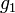$g_1$ and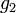$g_2$ are the two proportions,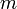$m$ and$n$ are their sample sizes and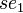$se_1$ and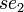$se_2$ are their standard errors:$t=\frac{g_1-g_2}{\sqrt{d_{eff}(se^2_1 + se^2_2)}}$,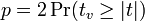$p = 2\Pr(t_v \ge |t|)$,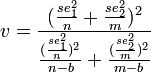$v = \frac{(\frac{se^2_1}{n} +\frac{se^2_2}{m} )^2}{\frac{(\frac{se^2_1}{n})^2}{n-b}+\frac{(\frac{se^2_2}{m})^2}{m-b} }$, or, if Weights and significance is set to Un-weighted sample size in tests (see Weights, Effective Sample Size and Design Effects),$v = n + m - 2$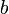$b$ is 1 if Bessel's correction is selected for Proportions in Statistical Assumptions and 0 otherwise.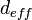$d_{eff}$ is Extra Deff.